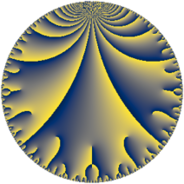# Properties

 Label 177.9.cLevel $177$ Weight $9$ Character orbit 177.c Rep. character $\chi_{177}(58,\cdot)$ Character field $\Q$ Dimension $80$ Newform subspaces $1$ Sturm bound $180$ Trace bound $0$

# Learn more about

## Defining parameters

 Level: $$N$$ $$=$$ $$177 = 3 \cdot 59$$ Weight: $$k$$ $$=$$ $$9$$ Character orbit: $$[\chi]$$ $$=$$ 177.c (of order $$2$$ and degree $$1$$) Character conductor: $$\operatorname{cond}(\chi)$$ $$=$$ $$59$$ Character field: $$\Q$$ Newform subspaces: $$1$$ Sturm bound: $$180$$ Trace bound: $$0$$

## Dimensions

The following table gives the dimensions of various subspaces of $$M_{9}(177, [\chi])$$.

Total New Old
Modular forms 162 80 82
Cusp forms 158 80 78
Eisenstein series 4 0 4

## Trace form

 $$80q - 10240q^{4} + 160q^{7} + 174960q^{9} + O(q^{10})$$ $$80q - 10240q^{4} + 160q^{7} + 174960q^{9} - 22680q^{12} - 59616q^{15} + 1199848q^{16} - 10608q^{17} - 27516q^{19} - 146436q^{20} - 974696q^{22} + 5718040q^{25} - 797484q^{26} - 3133000q^{28} + 1725924q^{29} + 4318800q^{35} - 22394880q^{36} - 732180q^{41} + 22752084q^{46} + 8703936q^{48} + 55899176q^{49} - 10373832q^{51} - 39265944q^{53} - 11408040q^{57} - 33575112q^{59} - 18034488q^{60} + 13038600q^{62} + 349920q^{63} - 241654260q^{64} - 35711928q^{66} + 36772608q^{68} - 235272660q^{71} - 63050712q^{74} + 74363184q^{75} + 9454680q^{76} - 10865988q^{78} + 17252580q^{79} + 318203976q^{80} + 382637520q^{81} - 20743128q^{84} - 27245820q^{85} + 105666984q^{86} + 29437992q^{87} + 82079788q^{88} + 121215992q^{94} - 690837276q^{95} + O(q^{100})$$

## Decomposition of $$S_{9}^{\mathrm{new}}(177, [\chi])$$ into newform subspaces

Label Dim. $$A$$ Field CM Traces $q$-expansion
$$a_2$$ $$a_3$$ $$a_5$$ $$a_7$$
177.9.c.a $$80$$ $$72.106$$ None $$0$$ $$0$$ $$0$$ $$160$$

## Decomposition of $$S_{9}^{\mathrm{old}}(177, [\chi])$$ into lower level spaces

$$S_{9}^{\mathrm{old}}(177, [\chi]) \cong$$ $$S_{9}^{\mathrm{new}}(59, [\chi])$$$$^{\oplus 2}$$